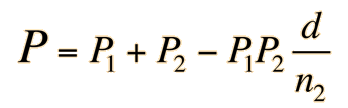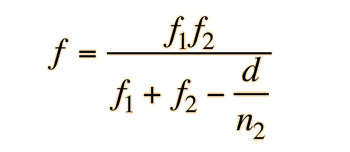# Gullstrand's Equation

Thick lenses can be handled with thin lens type equations if the distances are measured from hypothetical principal planes. The power of a lens with respect to the second principal plane H2 is given by Gullstrand's Equation:The focal length f with respect to that second principal plane is given by:Forms of Gullstrand's Equation are applicable to both separated lenses and to single thick lenses. Since Gullstrand powers and focal lengths are measured with respect to a hypothetical plane, it is often more useful to deal with front and back vertex focal lengths.

 Calculations
Index

Lens concepts

Reference
Meyer-Arendt
4th ed, Ch 3

 HyperPhysics***** Light and Vision R Nave
Go Back